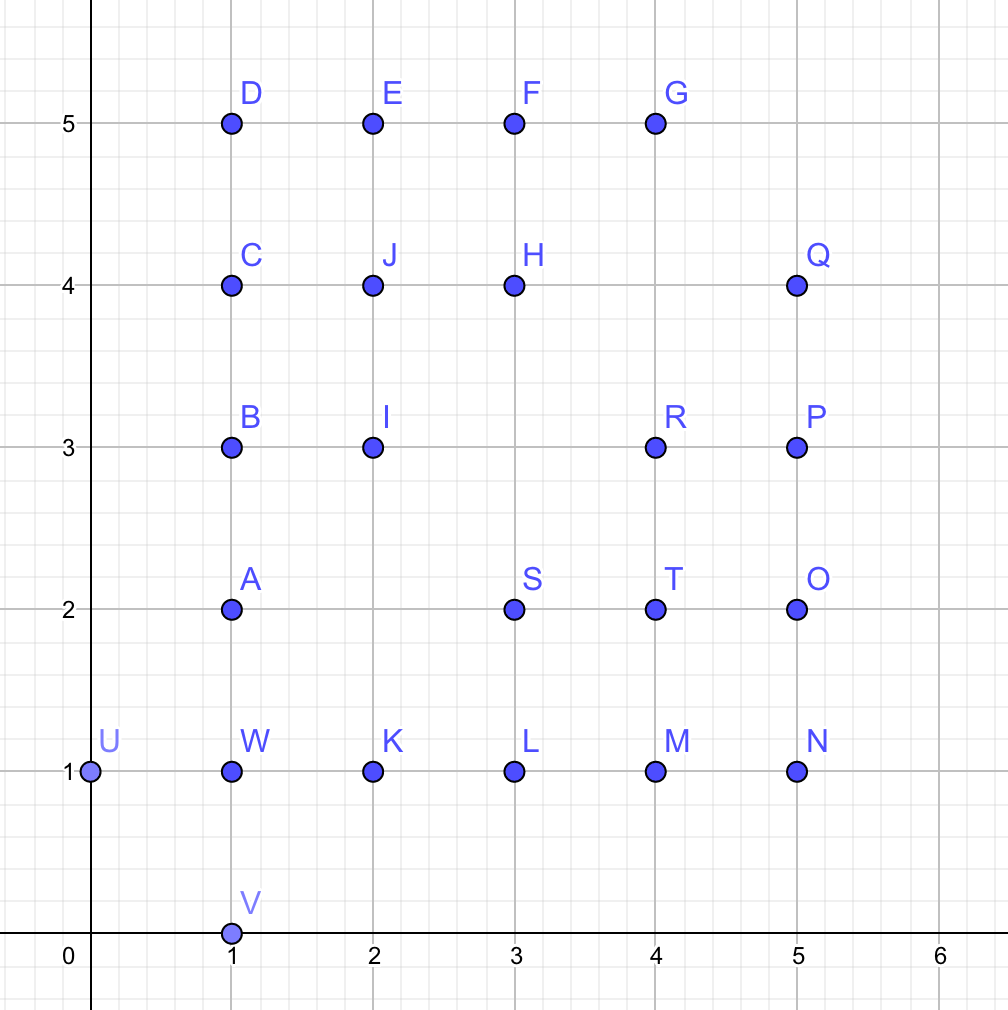# 题目描述【数据规模和约定】

# 解析$\text{ans}(n + 1) = 3 + 2\sum{x = 1}^{n} \sum{y = 1}^{x - 1}[\gcd(x, y) = 1]$
$= 3 + 2\sum{x = 1}^{n} (\sum{y = 1}^{x}[\gcd(x, y) = 1] - [\gcd(x, x) = 1])$
$= 3 + 2\sum_{x = 1}^{n} (\varphi(x) - [\gcd(x, x) = 1])$

（式子要分行写是因为我博客的 Math 引擎好像炸了。。。渲染不了多行，将就看吧/kk）